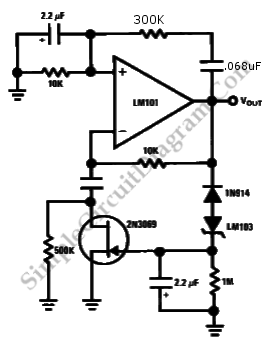# Wien Bridge Sine Wave Oscillator

Getting the right amplifier loop gain is the major problem in producing a low distortion constant amplitude sine wave. However, we can solve this problem with Wien bridge sine wave oscillator. Here is the circuit :This circuit uses the 2N3069 JFET as a voltage variable resistor in the amplifier feedback loop. The voltage reference for the peak sine wave amplitude is provided by the LM103 zener diode. It is fed and rectified to the 2N3069’s gate. So its loop gain and channel resistance are varied.

The peak output voltage is given by Vp =V2+1V
The frequency of this circuit is 10Hz. [Source: National Semiconductor Application Note]